# 条件随机场（conditional random field CRF）

## 核心点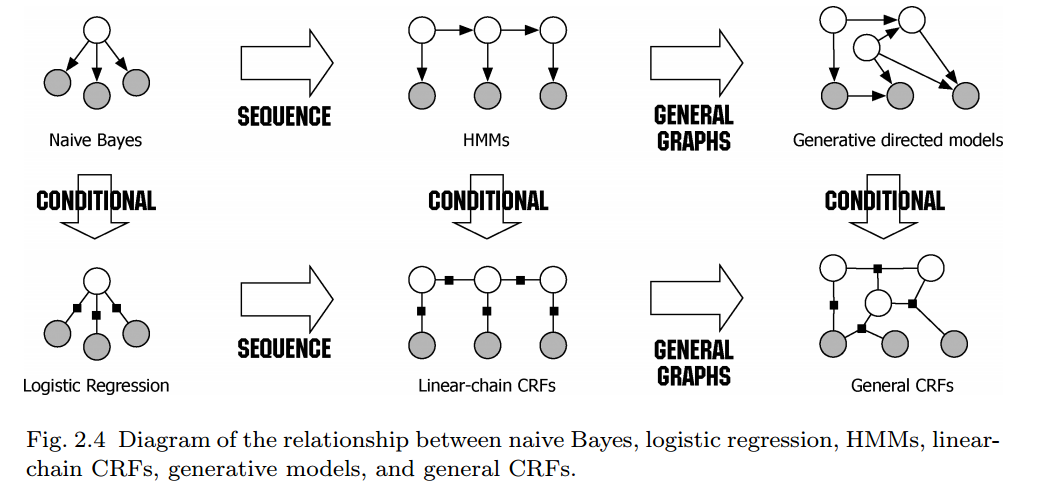• 成对马尔可夫性：设$u,v$是无向图$G$中任意两个没有边连接的结点，其他所有结点表示为$O$，对应的随机变量分别用$Y_u,Y_v,Y_O$表示，成对马尔可夫性是指给定随机变量组$Y_O$的条件下随机变量$Y_u,Y_v$是条件独立的，如下：$$P(Y_u,Y_v|Y_O)=P(Y_u|Y_O)P(Y_v|Y_O)$$
• 局部马尔可夫性：设$v\in V$$G$中任意一个节点，$W$是与$v$有边连接的所有节点，$O$$v$$W$以外的其他所有节点。$v$表示的随机变量是$Y_v$$W$表示的随机变量是$Y_w$$O$表示的随机变量是$Y_o$。局部马尔可夫性是在给定随机变量组$Y_w$的条件下随机变量$Y_v$与随机变量$Y_o$是独立的。$$P(Y_v,Y_O|Y_W)=P(Y_v|Y_w)P(Y_O|Y_W)$$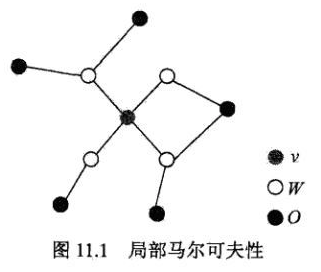• 全局马尔可夫性：$A,B$$G$中被C分开的任意节点集合。全局马尔科夫性是指给定$Y_c$条件下$Y_A$$Y_B$是条件独立的，即$$P(Y_A,Y_B|Y_C)=P(Y_A|Y_C)P(Y_B|Y_C)$$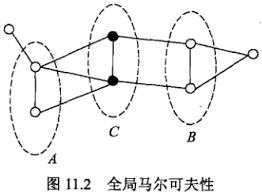## 线性链条件随机场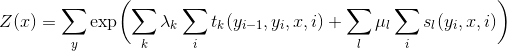$Z(x)$作为规范化因子，是对$y$的所有可能取值求和。我们可以用下图来理解：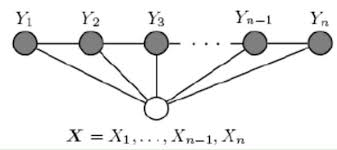• 转移特征$t_k(y_{i−1},y_i,x,i)$是定义在边上的特征函数（transition），依赖于当前位置$i$和前一位置$i-1$；对应的权值为$λ_k$
• 状态特征$s_l(y_i,x,i)$是定义在节点上的特征函数（state），依赖于当前位置$i$；对应的权值为$μ_l$

## 简化形式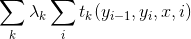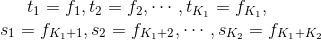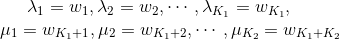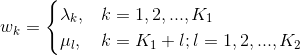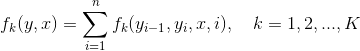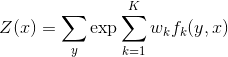# CRF分词

• 句子$S$（就是我们要标注词性的句子）
• $i$，用来表示句子$S$中第$i$个单词
• $l_i$，表示要评分的标注序列给第i个单词标注的词性
• $l_{i-1}$，表示要评分的标注序列给第$i-1$个单词标注的词性

Note：在实际的应用时，除了单一的特征选取，我们通常会通过构造复合特征的方式，考虑更多的上下文信息。

# CRF VS HMM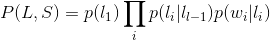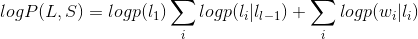• HMM是生成模型以联合概率建模，CRF是判别模型以条件概率建模
• HMM为了简化计算做了有限状态假设和一对一假设（当前的单词只依赖于当前的标签，当前的标签只依赖于前一个标签），所以在特征选取上会有很多限制；而CRF可以定义数量更多，种类更丰富的特征函数（如定义一个特征函数f，考虑当前词离句首的距离，来着眼于整个句子）。
• CRF可以使用任意的权重 将对数HMM模型看做CRF时，特征函数的权重由于是log形式的概率，所以都是小于等于0的，而且概率还要满足相应的限制，但在CRF中，每个特征函数的权重可以是任意值，没有这些限制。

Note:其实在HMM和CRF之间还有一个MEMM，即最大熵马尔科夫模型，MEMM模型是对转移概率和表现概率建立联合概率，统计时统计的是条件概率，但MEMM容易陷入局部最优，是因为MEMM只在局部做归一化（标记偏置问题），不过一般用的不多我们就不过多介绍，可参考

# 代码实现

## 实验结果

• Label sequences
1000 sequences labeled 3.96%/43.30%
2000 sequences labeled 3.89%/44.00%
3000 sequences labeled 3.87%/44.50%
Nb sequences : 3985
Token error : 3.85%
Sequence error: 44.74%
• Per label statistics
B Pr=0.96 Rc=0.98 F1=0.97
E Pr=0.96 Rc=0.98 F1=0.97
S Pr=0.97 Rc=0.95 F1=0.96
M Pr=0.92 Rc=0.87 F1=0.90
• Done

# 参考文献

1. HMM MEMM CRF 区别 联系
2. 如何轻松愉快地理解条件随机场（CRF）？
3. NLP —— 图模型（二）条件随机场（Conditional random field，CRF）
4. An Introduction to Conditional Random Fields
5. HMM MEMM CRF 区别 联系
6. 《统计学习方法》 李航
posted @ 2018-12-28 12:16  xlturing  阅读(5865)  评论(0编辑  收藏  举报